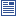Accessibility options:

# Pythagoras' theorem and applications to basic problems resources

Show me all resources applicable to

### Motivating Mathematics (1)The pizza on the hypotenuse
This mathtutor extension video illustrates Pythagoras's theorem with pizzas. This resource is released under a Creative Commons license Attribution-Non-Commercial-No Derivative Works and the copyright is held by Skillbank Solutions Ltd.

### Quick Reference (1)Pythagoras' theorem
This leaflet reminds students of Pythagoras' theorem and gives some simple examples. (Engineering Maths First Aid Kit 4.5)

### Teach Yourself (1)Pythagoras' theorem
This unit states Pythagoras' theorem and illustrates its use in the solution of two- and three-dimensional problems.

### Test Yourself (1)Maths EG
Computer-aided assessment of maths, stats and numeracy from GCSE to undergraduate level 2. These resources have been made available under a Creative Common licence by Martin Greenhow and Abdulrahman Kamavi, Brunel University.

### Third Party Resources (1)University of East Anglia (UEA) Interactive Mathematics and Statistics Resources
The Learning Enhancement Team at the University of East Anglia (UEA) has developed la series of interactive resources accessible via Prezi mind maps : Steps into Numeracy, Steps into Algebra, Steps into Trigonometry, Bridging between Algebra and Calculus, Steps into Calculus, Steps into Differential Equations, Steps into Statistics and Other Essential Skills.

### Video (3)Hyperbolic functions - David Saunders
This mathtutor extention video explains the derivation of hyperbolic functions starting from two-dimensional space. This resource is released under a Creative Commons license Attribution-Non-Commercial-No Derivative Works and the copyright is held by Skillbank Solutions Ltd.Pythagoras' theorem
Pythagoras theorem - the square on the hypotenuse is equal to the sum of the squares on the other two sides - is well known. In this tutorial we revise the theorem and use it to solve problems in right-angled triangles. A less familiar form of the theorem is also considered. (Mathtutor Video Tutorial) This resource is released under a Creative Commons license Attribution-Non-Commercial-No Derivative Works and the copyright is held by Skillbank Solutions Ltd.Pythagoras' Theorem - an Animation
This mathtutor animation shows visually that the square on the hypotenuse is equal to the sum of the squares on the other two sides. This resource is released under a Creative Commons license Attribution-Non-Commercial-No Derivative Works and the copyright is held by Skillbank Solutions Ltd.

### Workbook (2)Euclidean Geometry
Designed for self-study, this drawing-led introduction to the geometry of Euclid takes the learner from first principles through to constructions and mathematical proofs as well as covering practical applications of the techniques learnt in art and design. It concludes with a study of the pentagon, golden ratio and their surprising mathematical interconnection. The resources comprise a 100-page booklet and supporting interactive resources. These resources have been created by Rich Cochrane and Andrew McGettigan (Central Saint Martins, UAL) and reviewed by Prof Jeremy Gray (Open University). They were funded by a sigma Resource Development grant and contributed to the mathcentre Community Project.Euclidean Geometry - interactive resources
This zip file contains supporting interactive resources to accompany the self-study booklet "Euclidean Geometry". These resources have been created by Rich Cochrane and Andrew McGettigan (Central Saint Martins, UAL) and reviewed by Prof Jeremy Gray (Open University). They were funded by a sigma Resource Development grant and contributed to the mathcentre Community Project.

Website design by Pink Mayhem, Leicester